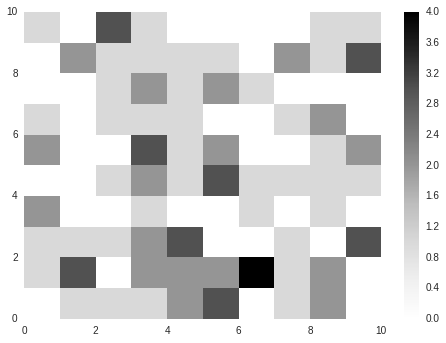# Basic Grid¶

In this notebook, we'll explore one of the most common types of environment - a grid. In this case, we'll be creating a two-dimension (2D) square grid. It's important to remember that grids can take other forms, including:

• one-dimensional
• three-dimensional
• hexagonal
• triangular

2D grids are the simple to implement and understand, and they are a very common choice for real-world spatial models. Let's begin by importing some libraries and exploring how a grid works.

### Imports

The imports below provide us with:

• numpy: numerical python utilities, including multi-dimensional arrays/matrices
• matplotlib.pyplot: plotting library

In addition, the %matplotlib inline statement ensures that our plots are visible in ipython.

In :
%matplotlib inline
# Imports
import numpy
import matplotlib.pyplot as plt

import seaborn; seaborn.set()


## Grids terminology and notation¶

Before we begin, it's important to agree our terminology and notation down. Below are some grid terms that we'll be using:

• cell: a region of the grid bounded by sides that can be identified by a position
• lattice: synonym for grid (specially, integer lattice)
• neighbors: cells that are adjacent to a given reference cell
• Moore neighborhood: a definition of cell adjacency that includes all touching cells• von Neumann neighborhood: a definition of cell adjacency that includes only unit-distance cells, i.e., along cardinal directions• Manhattan distance: also known as taxicab or $\ell_1$ distance, Manhattan distance is the metric used in calculating von Neumann neighborhoods

## Grids with Numerical Values¶

Now that we know how to refer to elements of our grid, let's see the most common way to store and display grids with numerical values. These are grids whose cells store a numerical value, e.g., :

• integer ID of an agent; e.g., cell (3,4) contains agent #17
• integer population size; e.g., number of people living in a certain neighborhood
• decimal quantity; e.g., mass of vegetation per square meter

To store this information, we can use numpy.array objects. To brush up on arrays, you might review the following links:

• Numpy basics

• Numpy tutorial

### Creating an empty array¶

To start, let's see how to create an empty array. We can do this in a number of ways, including:

• NaN: create an array whose cells are filled with NaN ("not-a-number")
• zero: create an array whose cells are filled with zeros

Depending on the interpretation of cell values, your appropriate choice may vary.

#### Creating an empty array: NaN¶

In :
# Set the grid size
grid_size = 5

# Create the grid
space = numpy.full((grid_size, grid_size), numpy.nan)
print(space)

[[ nan  nan  nan  nan  nan]
[ nan  nan  nan  nan  nan]
[ nan  nan  nan  nan  nan]
[ nan  nan  nan  nan  nan]
[ nan  nan  nan  nan  nan]]


#### Creating an empty array: zero¶

In :
# Set the grid size
grid_size = 5

# Create the grid
space = numpy.zeros((grid_size, grid_size))
print(space)

[[ 0.  0.  0.  0.  0.]
[ 0.  0.  0.  0.  0.]
[ 0.  0.  0.  0.  0.]
[ 0.  0.  0.  0.  0.]
[ 0.  0.  0.  0.  0.]]


#### Visualizing our grid¶

Our next step should be to visualize the grid in a more scalable fashion than reviewing the full array of cell values. Instead, we can visualize the grid in a form that allows our visual cortex to provide context and characterization.

Let's start by visualizing our "zero-d" space using the matplotlib.pyplot method pcolor.

In :
# Now show the space
plt.figure()
plt.pcolor(space, snap=True)
plt.colorbar()

Out:
<matplotlib.colorbar.Colorbar at 0x782b8d0>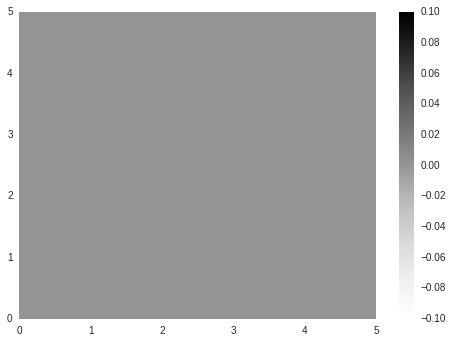Of course, since this is an "empty" grid with no values, we don't see much. Let's manually set one of the cells from 0 to 1 and plot again.

In :
# Change cell (1,1)'s value to 1
space[1, 1] = 1

# Now show the space
plt.figure()
plt.pcolor(space, snap=True)
plt.colorbar()

Out:
<matplotlib.colorbar.Colorbar at 0x7be8510>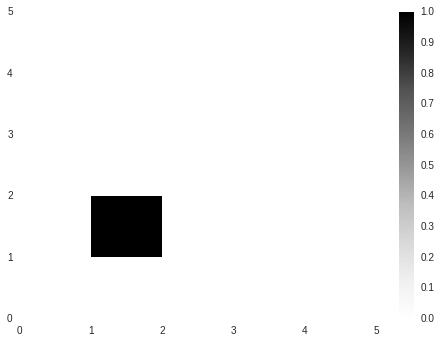There are two things to note:

• cell (1,1) corresponds to the second row and second column in the grid because we are using zero-indexing
• (0, 0) is in the lower left corner for this visualization; not all visualizations may choose this orientation! For example, many follow a convention like words on a page, with (0, 0) in the upper left corner

### Initializing a grid with random values¶

Grids are commonly setup with initial conditions, including:

• endowments of timber or other resources
• populations per square mile or other densities
• agent positions

Let's walk through creating a grid that represents the binary presence of a resource. Our parameters are as follows:

• grid_size: "dimension" of the grid, i.e., number of cells in each row or column

• num_cells: number of cells to sample for resource endowment

In order to determine which cells to endow, we'll need to sample from a random distribution. If you haven't already, now would be a great time to review the Basic Distributions notebook in the basic-random folder. We'll make the following assertions for our spatial distribution of resource:

• the row coordinate will be uniformly distributed across the entire discrete grid
• the column coordinate will be uniformly distributed across the entire discrete grid
• the row and column coordinates will be drawn independently from each other
In :
# Set the grid and cell parameters
grid_size = 25
num_cells = 10

# Create the space and activate random cells
space = numpy.zeros((grid_size, grid_size))

# Now sample the agents.
for cell_id in range(num_cells):
# Sample random position
row = numpy.random.randint(0, grid_size)
col = numpy.random.randint(0, grid_size)

# "Endow" the cell with the resource by setting its value to 1.
space[row, col] = 1

# Output some info about the agent.
print("Endowing cell ({0}, {1}).".format(row, col))

# Now show the space
plt.figure()
plt.pcolor(space, snap=True)
plt.colorbar()

Endowing cell (4, 20).
Endowing cell (5, 16).
Endowing cell (2, 16).
Endowing cell (5, 11).
Endowing cell (1, 14).
Endowing cell (5, 10).
Endowing cell (12, 0).
Endowing cell (6, 5).
Endowing cell (19, 15).
Endowing cell (15, 12).

Out:
<matplotlib.colorbar.Colorbar at 0x7fc0f90>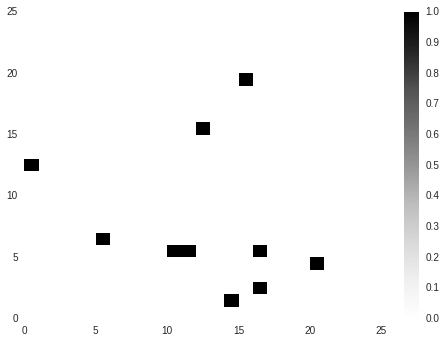## What have we done?¶

You should now see a small grid with blank background and a number of endowed cells.

### Questions¶

1. Are we guaranteed to always have num_cells cells with endowment? Why or why not?
2. How can the spatial distribution be characterized?

## Slightly more complicated example¶

Let's try a slightly more complicated example by making the following complications:

• Choose a more interesting spatial distribution.
• Represent the quantity, not just presence, of a resource
In :
# Set the grid and agent parameters
grid_size = 25
num_cells = 10

# Create the space and activate random cells
space = numpy.zeros((grid_size, grid_size))

# Now sample the agents.
for cell_id in range(num_cells):
# Sample random position
row = int(numpy.random.normal((grid_size) / 2.0, spread))
col = int(numpy.random.normal((grid_size) / 2.0, spread))

# "Activate" the cell by setting its value to 1.
space[row, col] += 1

# Output some info about the agent.
print("Endowing cell ({0}, {1}).".format(row, col))

# Now show the space
f = plt.figure()
plt.pcolor(space, snap=True)
plt.colorbar()

Endowing cell (13, 11).
Endowing cell (13, 12).
Endowing cell (13, 13).
Endowing cell (12, 12).
Endowing cell (10, 14).
Endowing cell (12, 12).
Endowing cell (11, 13).
Endowing cell (11, 13).
Endowing cell (13, 12).
Endowing cell (12, 12).

Out:
<matplotlib.colorbar.Colorbar at 0x84efb90>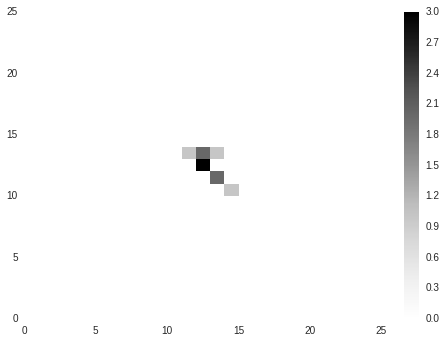## What have we done (this time)?¶

You should now see a small grid with blank background and a number of endowed cells. Unlike the previous example, the intensity of these cells should vary with the quantity of resource that has been placed.

### Questions¶

1. How can the spatial distribution be characterized? How does the spread parameter come into play?
2. What is the range of possible values for cell quantities?

## Interactive plotting¶

Next, let's create an interactive widget that helps us understand how the spread parameter affects the grid's initial conditions. In the code below, we wrap our example above in a method labeled gaussian_initial_grid, which we then call via the interact method.

When you run the code, perform the following visual "experiments":

• Fixing num_cells and spread, vary grid_size. Does the qualitative character of the initial conditions change?
• Fixing grid_size and spread, vary num_cells. Does the qualitative character of the initial conditions change?
• Fixing num_cells and grid_size, vary spread. Does the qualitative character of the initial conditions change?
In :
# First, we'll wrap our experiments above in a method that takes as input our grid parameters.
"""
Create an initial 2D grid with Gaussian/normal-distributed cells.
"""
# Create the space and activate random cells
space = numpy.zeros((grid_size, grid_size))

# Now sample the agents.
for cell_id in range(num_cells):
# Sample random position
row = int(numpy.random.normal((grid_size) / 2.0, spread))
col = int(numpy.random.normal((grid_size) / 2.0, spread))

# "Activate" the cell by setting its value to 1.
space[row, col] += 1

# Now show the space
f = plt.figure()
plt.pcolor(space, snap=True)
plt.colorbar()

# Import widget methods
from IPython.html.widgets import interact, fixed

interact(gaussian_initial_grid, grid_size=(5, 100),
num_cells = (1, 500),

Out:
<function __main__.gaussian_initial_grid>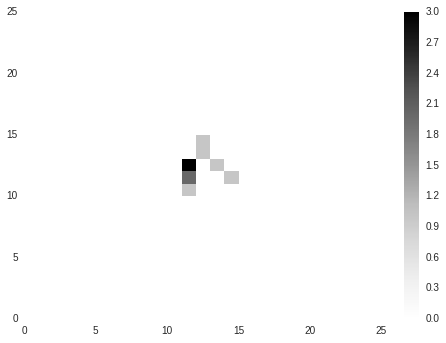## Boundaries¶

If all went as planned, in your third experiment with spread, you should have generated an error. This particular error is called an IndexError, because it occured when we tried to access or index a cell that did not exist in our space.

For example, if we have a (10, 10) grid, there are no cells with the label (27, 4) or (-15, 8). However, based on our method for sampling the resource positions, there is some non-zero probability that we will try these cells.

This is an example of boundary in space. There are a number of approaches to addressing it:

• change assumptions about distributions or dynamics so that the environment terminates; for example, we could:
• clump all resources up at the edges; for example, if we drew -4 as a column, place the resource instead in column 0
• change to a distribution that allows for bounded support, i.e., a uniform distribution or truncated normal
• wrap cells around the boundary, similar to a torus or sphere; for example, if we drew -1 as a column, we would wrap all the way around to the last column and place the resource there instead.

In the example below, we'll go with the wrapping or torus approach. To obtain the desired effect, we'll use the modulo operator %.

In :
# First, we'll wrap our experiments above in a method that takes as input our grid parameters.
"""
Create an initial 2D grid with Gaussian/normal-distributed cells with wrapping.
"""
# Create the space and activate random cells
space = numpy.zeros((grid_size, grid_size))

# Now sample the agents.
for cell_id in range(num_cells):
# Sample random position
row = int(numpy.random.normal((grid_size) / 2.0, spread)) % grid_size
col = int(numpy.random.normal((grid_size) / 2.0, spread)) % grid_size

# "Activate" the cell by setting its value to 1.
space[row, col] += 1

# Now show the space
f = plt.figure()
plt.pcolor(space, snap=True)
plt.colorbar()

# Import widget methods
from IPython.html.widgets import interact, fixed

interact(gaussian_initial_grid, grid_size=(5, 100),
num_cells = (1, 500),

<function __main__.gaussian_initial_grid>